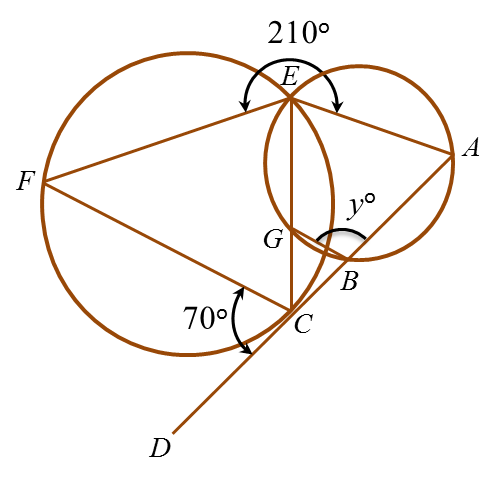# 8.4.1 Circles III, Paper 1 (Short Questions)

Question 1:In figure above, FAD is a tangent to the circle with centre O. AEB and OECD are straight lines. The value of y is

Solution:

AOD= 180o  – 90o – 34o= 56o
y = 56o  ÷ 2 = 28o

Question 2:In figure above, PQR is a tangent to the circle QSTU at and TUPV is a straight line. The value of y is

Solution:

QTS = ∠RQS = 40o
SQT= ∠QTS = 40o (isosceles triangle)
PQT= 180o  – 40o – 40o= 100o
TPQ= 180o  – 115o = 65o
y = 180o  – 100o – 65o= 15o

Question 3:In figure above, ABC is a tangent to the circle BDE with centre O, at B.
Find the value of y.

Solution:
BOD= 2 × ∠BED
= 2 × 35o = 70o
ODB = ∠OBD
= (180– 70o÷ 2 = 55o

EDB = ∠ EBA = 75o
yo + ∠ ODB = 75o
yo + 55o = 75o
y = 20o

Question 4:In figure above, ABCD is a tangent to the circle CEF at point C. EGC is a straight line. The value of y is

Solution:
$\begin{array}{l}\angle CEF=\angle DCF={70}^{\circ }\\ \angle AEG+{70}^{\circ }+{210}^{\circ }={360}^{\circ }\\ \angle AEG={80}^{\circ }\\ \\ \text{In cyclic quadrilateral}ABGE,\\ \angle ABG+\angle AEG={180}^{\circ }\\ {y}^{\circ }+{80}^{\circ }={180}^{\circ }\\ y=100\end{array}$

### 1 thought on “8.4.1 Circles III, Paper 1 (Short Questions)”

1.Hi, could you please provide more clarity for Q1? Why do i need to divide AOD by 2 to get y?